eMath Studio overview

Structured Derivations in 4f Studio

4f Studio provides an efficient and convenient environment for teaching mathematics using structured derivations.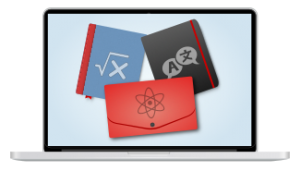4f Studio as the learning environment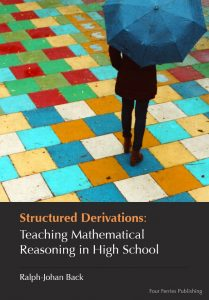Tutorials and textbooks available in 4f  Studio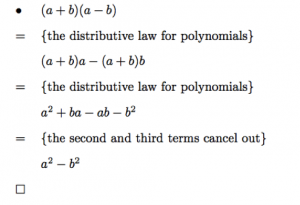SD-editor included in  4f Studio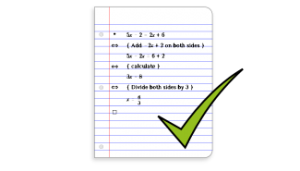4f Checker available in 4f  Studio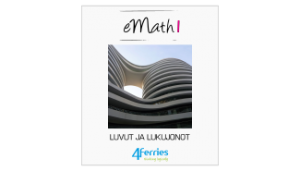eMath textbooks can be used in 4f  Studio courses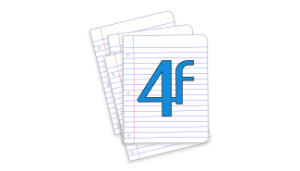All 4f  Studio mathematics tools available i 4f Studio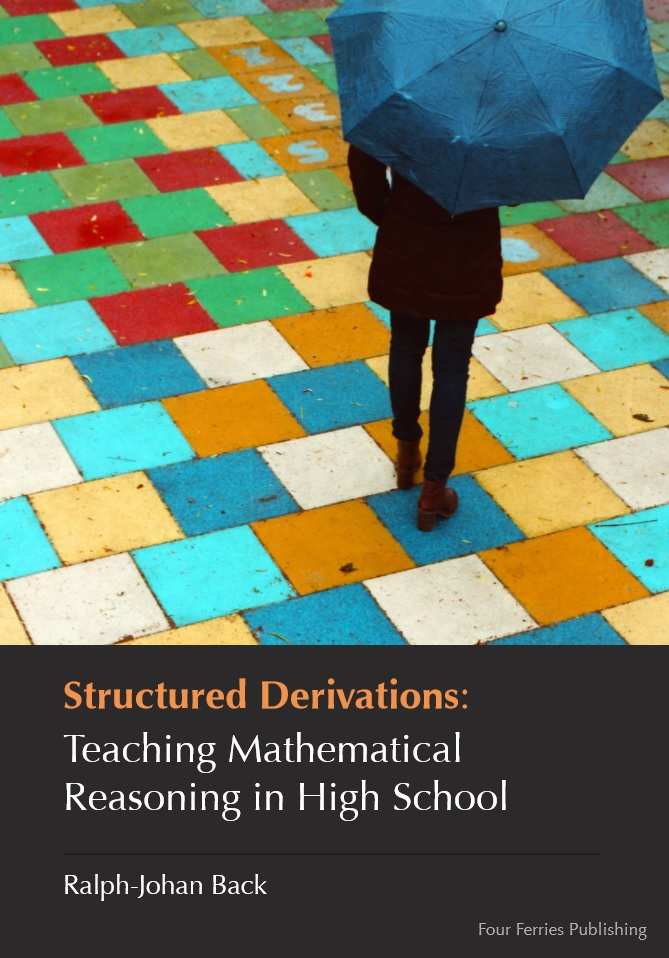Structured Derivations

Structured derivations make the structure of mathematical reasoning clearly visible. The verbal justifications required for each derivation step helps the reader to understand why each step is correct. Structured derivations make mathematical reasoning much easier to understand, compared to the standard way of presenting mathematical arguments.

SD Editor

The SD editor is used to write structured derivations. It will take care of the formatting so that you can concentrate on how to organize the mathematical reasoning.

The editor shows you the editing operations that are allowed at each place in the derivation. The editor uses standard mathematical formula notation in the derivation. Mathematical symbols can be written using LaTeX shortcuts, or by picking the special symbols from a palette.

When making a new calculation step, the editor first creates a copy of the previous term. This makes it easy to make small changes in large formulas, and reduces typing errors in the derivation. Read more ...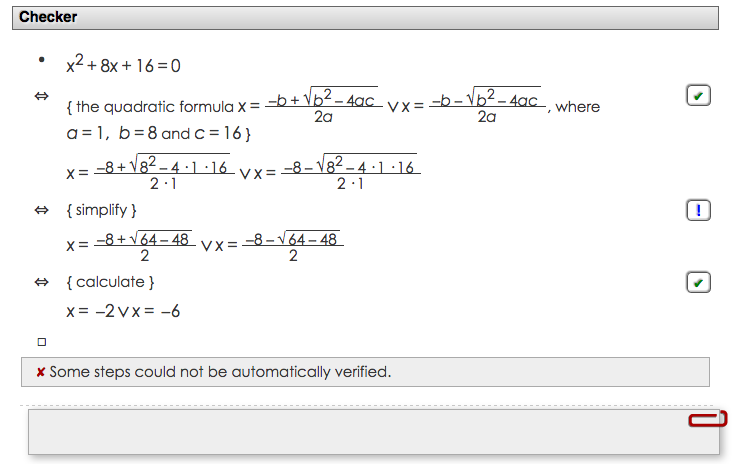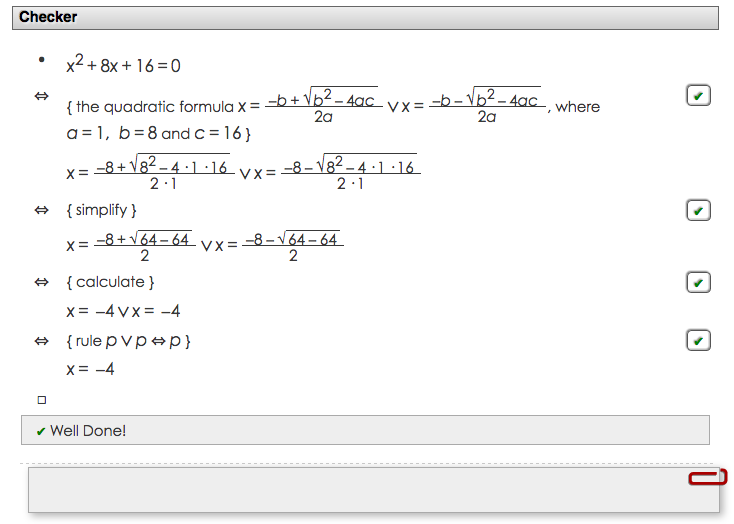Automatic Checking

The eMath checker reads a structured derivation step by step, and checks that each step is mathematically correct. It does this by expressing the correctness of the step as a mathematical theorem, and then using automatic theorem provers to try to prove the theorem. The checker warns the students for each step that has not been proved correct.  This helps students find errors in their own derivations, and tells them when they have a correct solution. Read more ...

eMath Textbooks

The eMath textbookseMath 1 - eMath 10,  cover all topics in high school mathematics (grades 10 - 12). The books are structured according to the Finnish national curriculum for high school math, but can be used also as references in other national curriculum,  the content of high school math is more or less the same all over the world. The books are available in three languages, Finnish, Swedish, and English. The books all use structured derivations for presenting different kinds of mathematical arguments, like proofs, calculations, geometric constructions, and general problem solving. The books contain a lot of assignments, which the students can solve directly in the book using the eMath editors available in 4f Studio. Read more...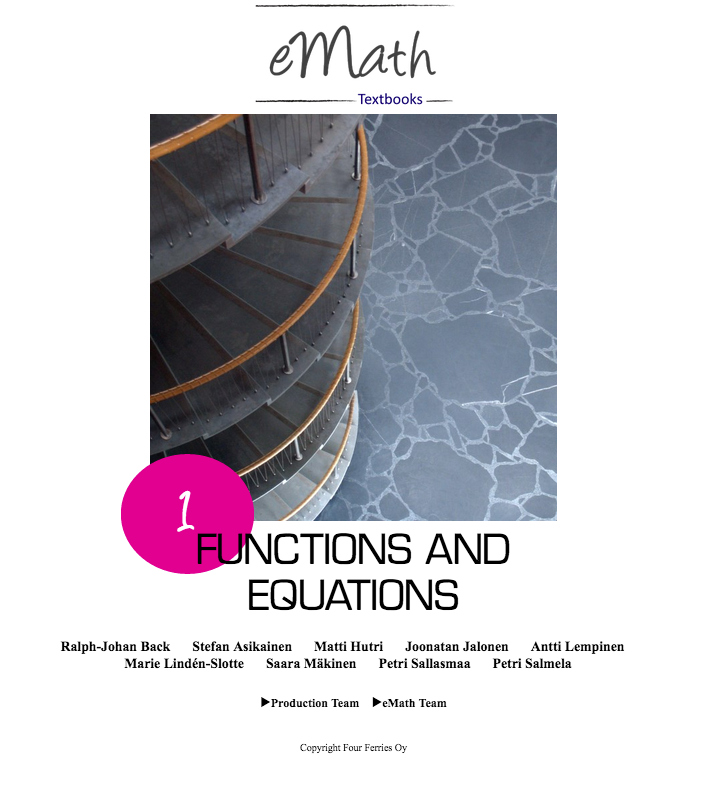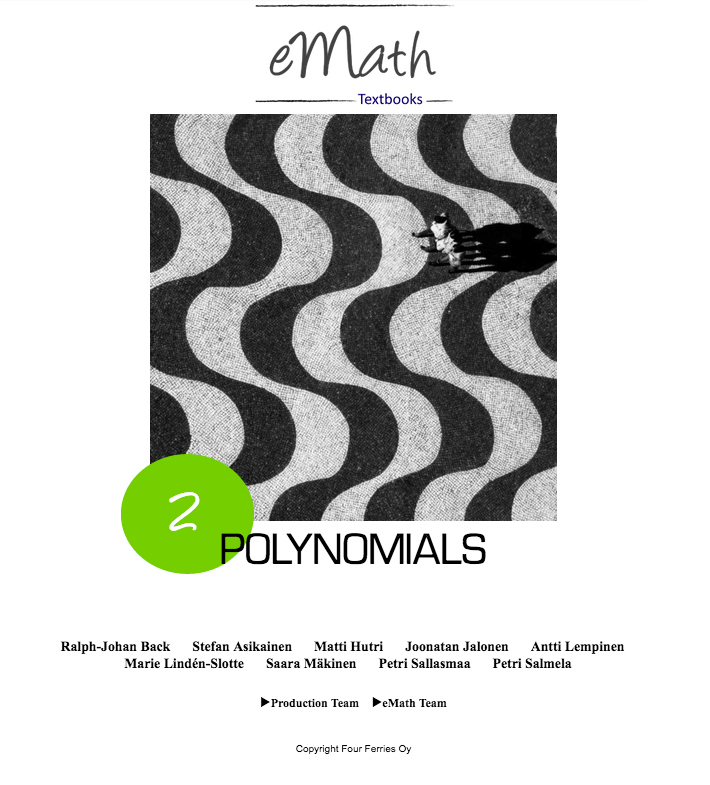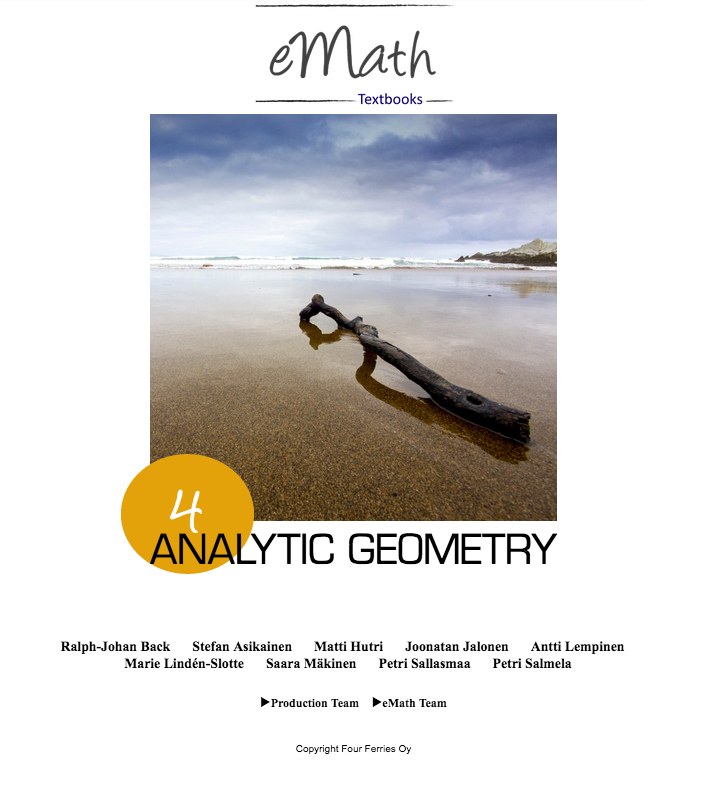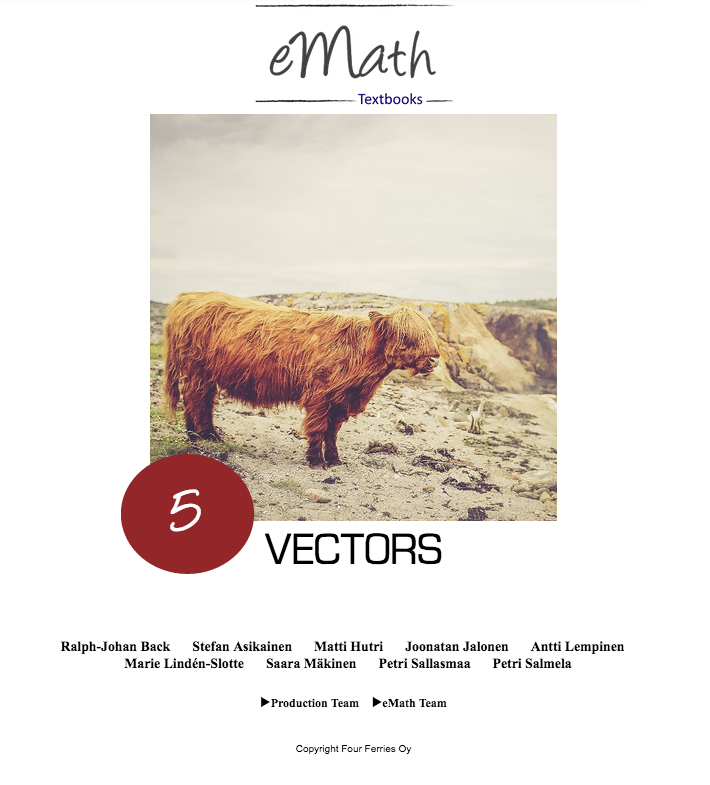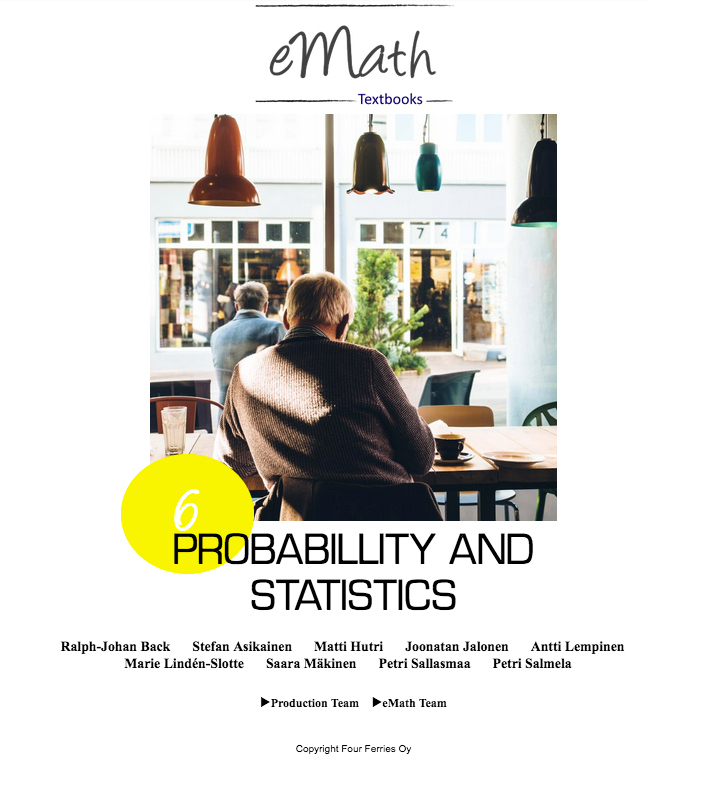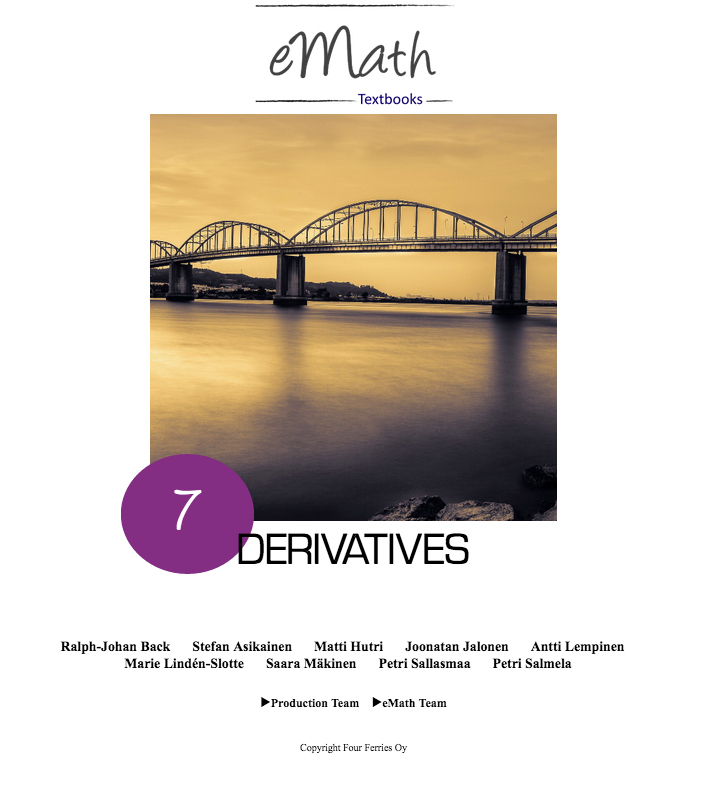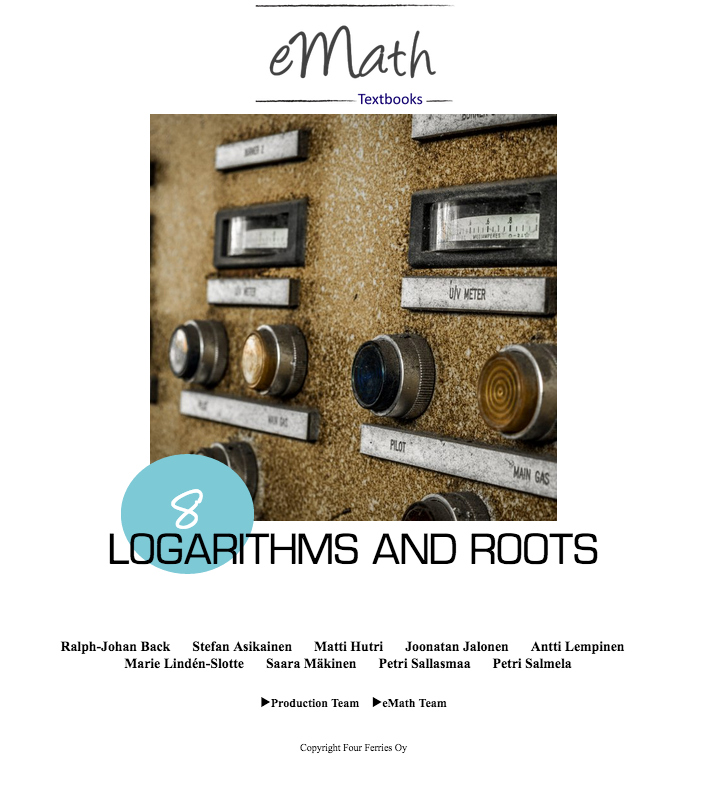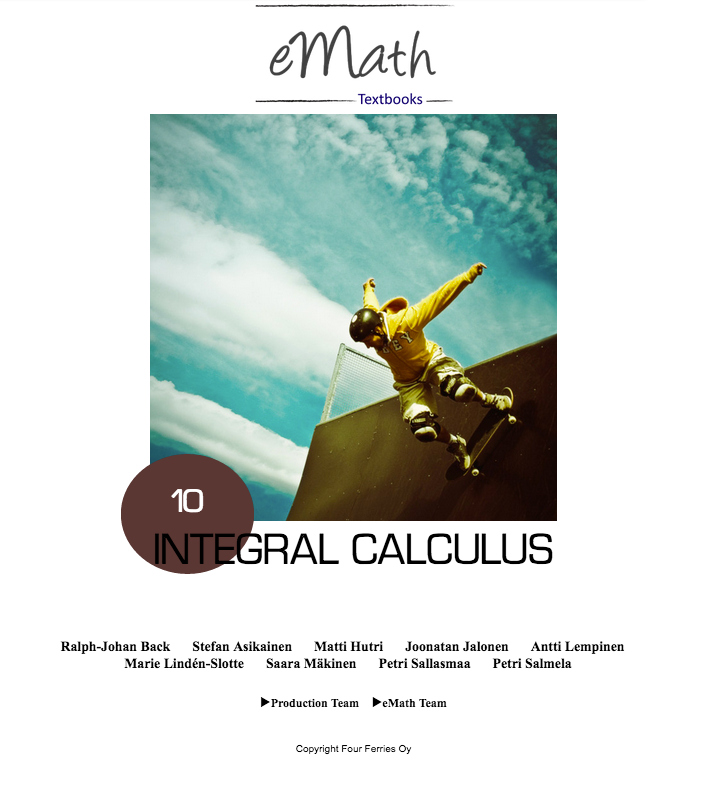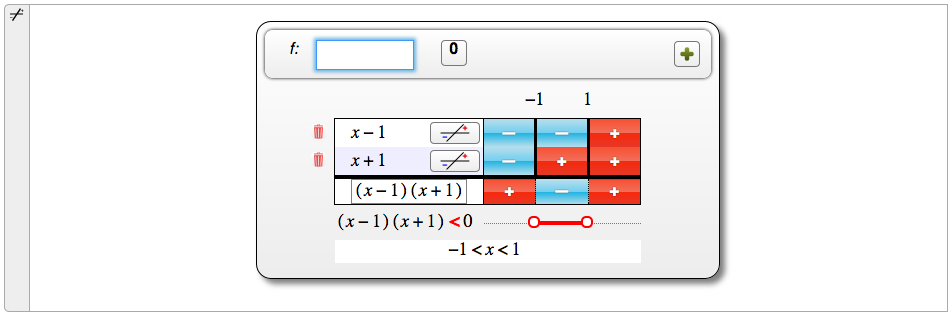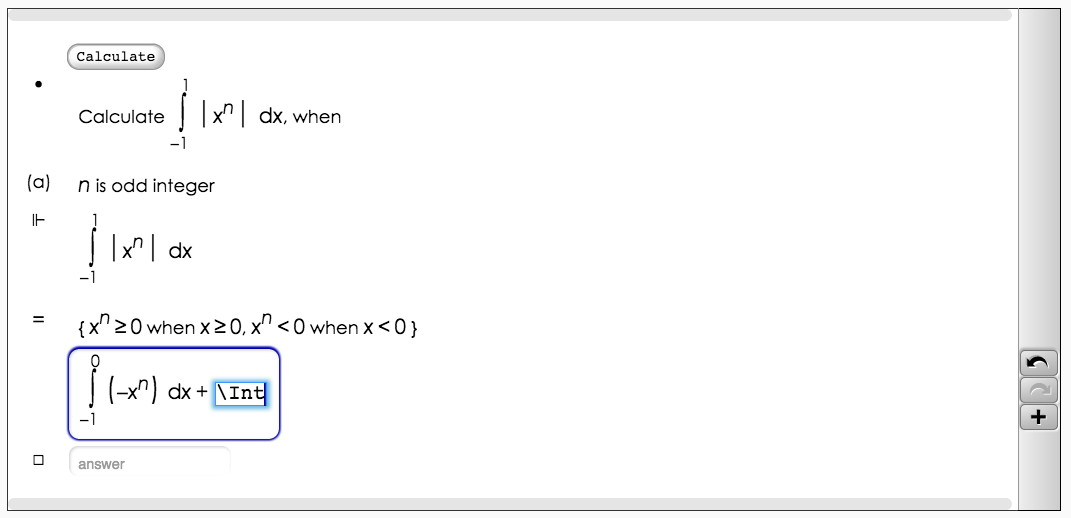4f Studio math tools

Using eMath textbooks and the math tools in 4f Studio provides a very powerful environment for mathematics education. eMath textbooks can be used as course books in 4f courses. The students can use 4f notebooks for creating mathematical content and use the notebook tools when solving assignments directly in the course book. Students can check the correctness of their solutions using the eMath Checker. The strong support for mathematical content elements makes 4f Studio particularly well suited for teaching mathematics using structured derivations. Read more ...